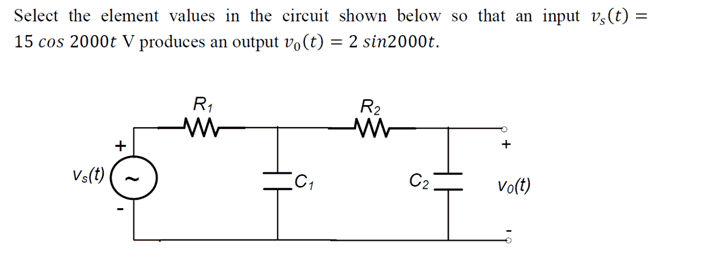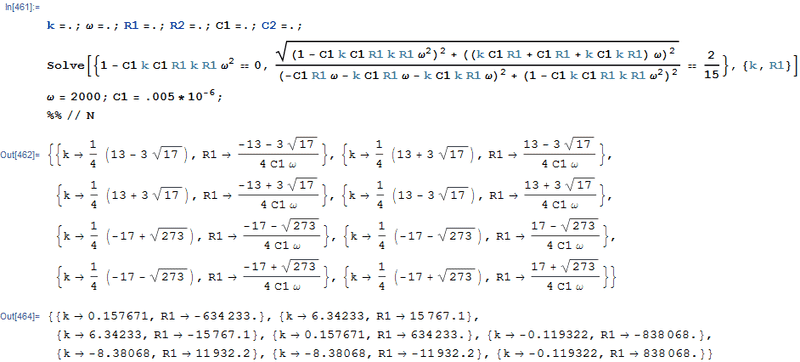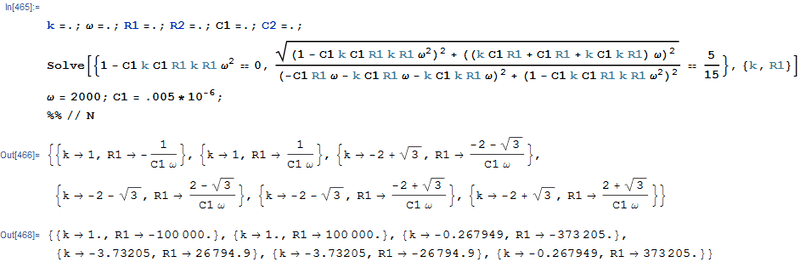# Calculating R and C values given input and output voltage

• Forcefedglas
In summary, the problem was to find the gain and angle of a filter with two identical stages. The solution involves transforming it to polar form and solving for values of R and θ.f

## Homework Statement## Homework Equations

$$V=IR$$

## The Attempt at a Solution

To simplify the algebra it was suggested that I set $$R_{1}=R_{2}=R$$ and $$C_{1}=C_{2}=C$$ So that's what I did. I labeled the voltage in the middle node as $$V_{1}$$Taking KCL at the middle node gives $$\frac{V_{s}-V_{1}}{R}-j\omega CV_{1}+\frac{V_{0}-V_{1}}{R} =0$$ which simplifies to $$V_{1}=\frac{V_{s}+V_{0}}{2+jR\omega C}$$Then I took KCL at the rightmost node, giving $$V_{0}=\frac{V_{1}}{1+jR\omega C}$$ after simplification. Substituting my previous expression for $$V_{1}$$ into the equation gives $$2+3jR\omega C-R^2\omega^2C^2=\frac{V_{s}+V_{0}}{V_{0}}$$After some more simplification. From here on I'm having trouble getting an answer out. I tried substituting in known values $$\omega=2000, V_{s}=15, V_{0}=-j2$$ and got some nonsensical equation with no solutions? I think the solution they (very briefly) explained involved transforming it to the polar form but I'm not seeing how that would simplify things. Any pointers will be greatly appreciated, thanks!

After some more simplification.
Are you able to arrange this into Vo / Vs =I'm not sure it's possible to solve this problem using the assumption of equal resistance and capacitance values. Essentially there are two filter stages of the same layout (simple low-pass RC stages). They will influence each other via their interface impedances, shifting their gains and phase angles. I suspect that you could design for the net phase angle or the gain, but not both at the same time. It would be a pretty nifty coincidence to find that a gain of 2/15 coincides with a phase shift of ##-\pi/2##.

If I may offer a suggestion? If you choose to make the input impedance of the second stage large compared to the output impedance of the first stage then they will effectively behave independently and then you can design each stage with its own gain and angle. The cascaded stages will then multiply gains and add phase angles.

Are you able to arrange this into Vo / Vs =$$\frac{1}{1-R^2\omega^2C^2+j3R\omega C}=\frac{V_{0}}{V_{s}}$$ or $$\frac{1-R^2\omega^2C^2-j3R\omega C}{(1-R^2\omega^2C^2)^2+9R^4\omega^4C^4}=\frac{V_{0}}{V_{s}}$$ after rationalization? I'm still having trouble seeing how to pull out a solution from here, though.

I'm not sure it's possible to solve this problem using the assumption of equal resistance and capacitance values. Essentially there are two filter stages of the same layout (simple low-pass RC stages). They will influence each other via their interface impedances, shifting their gains and phase angles. I suspect that you could design for the net phase angle or the gain, but not both at the same time. It would be a pretty nifty coincidence to find that a gain of 2/15 coincides with a phase shift of ##-\pi/2##.

If I may offer a suggestion? If you choose to make the input impedance of the second stage large compared to the output impedance of the first stage then they will effectively behave independently and then you can design each stage with its own gain and angle. The cascaded stages will then multiply gains and add phase angles.
I believe this problem was designed with that coincidence in mind. Thanks for the suggestion though, I'll try that method out as I still want to know how to solve this type of problem in a more general case.

$$\frac{1}{1-R^2\omega^2C^2+j3R\omega C}=\frac{V_{0}}{V_{s}}$$
(Will go with this, though I haven't checked your derivation...)
Next, express this in polar form: Vo/Vs = 1∠0° / A∠θ°

I believe this problem was designed with that coincidence in mind.
If that is the case then perhaps there's a typo in the question? I think would be solvable if the output had a magnitude of 5 rather than 2.

(Will go with this, though I haven't checked your derivation...)
Next, express this in polar form: Vo/Vs = 1∠0° / A∠θ°
$$\frac{V_{0}}{V_{s}} = \frac{1∠0°}{\sqrt{(1-R^2\omega^2C^2)^2+9(R\omega C)^2}∠arctan(\frac{3R\omega C}{1-R^2\omega^2C^2})}$$

If that is the case then perhaps there's a typo in the question? I think would be solvable if the output had a magnitude of 5 rather than 2.

At the start of class they suggested this method, and they described the solution very briefly at the end in a few seconds as there wasn't much time left. It's unlikely that no one in the class picked up on it at all but certainly possible I suppose.

Last edited:
(There's a missing power of 2 in the denominator.)

Vo/Vs can be written in polar form: you have the amplitude there, leave it as 1/... and the angle is the angle of the denominator but given with a negative sign.

Finally, equate this expression to the gain and angle implied in post #1 and solve for suitable values of R and C.

(There's a missing power of 2 in the denominator.)

Vo/Vs can be written in polar form: you have the amplitude there, leave it as 1/... and the angle is the angle of the denominator but given with a negative sign.

Finally, equate this expression to the gain and angle implied in post #1 and solve for suitable values of R and C.

Would equating angles imply $$arctan(\frac{3R\omega C}{1-R^2\omega^2 C^2})=90$$Meaning that $$R\omega C=1$$? Setting the amplitudes equal gives $$\omega^4R^4C^4+7R^2\omega^2C^2=56.25$$ which doesn't seem to give out values that agree with the first equation.

EDIT: The values do seem to work out when I set the magnitude equal to 5 as suggested by gneill meaning that there was infact either a typo in the question, or the markers also had the wrong answer down (and no one, including me, picked up on it), I'll have to double check with them either way. Out of curiosity why is it necessary to put a question like this in polar form in the first place? If the amplitude had been equal to 5 originally would the values have worked out in my original equation by equating the real and imaginary parts?

I appreciate all the help and I'll try out gneill's method when I have the time.

Last edited:
A practical general method might be to set the capacitor ratio, say, 1 or 10 or 0.1, and determine resistances according to specs.In your equation back in post #4, I think your 3 should be a 2.

The polar form is just easier to follow. You could stay with your post #4 complex expression, and use it.

I think the OP has got his solution, so here's how I did it.

As gneill says, there is no solution is we set R1=R2=R and C1=C2=C, with a gain of 2/15.

However, using his suggestion to have higher impedance in the second stage, a solution is possible.

There are only two equations possible, one for gain and one for phase angle. We need to find a way to formulate the problem so that only two unknowns are to be solved for.

A reasonable way to do this is to make R2 = k*R1 and C2 = k*C1. Then we can arbitrarily pick a value for either R1 or C1. I picked a value for C1 of .005 uF. Then we need only solve for k and R1.

Deriving an expression for the gain, and noting that the phase angle is -90 degrees when (1-R1 C1 R2 C2 ω2) = 0, we can set up two equations, one for phase angle of -90 degrees, and one for a gain of 2/15.

Using Mathematica to do the heavy lifting, I get several solutions, not all of which are feasible:So a solution with C1=.005 uF is k = 6.34233 and R1 = 15767.1

Testing the circuit with these values verifies its correctness.

Interestingly, if the gain is set to 5/15, these solutions result. Notice that k is exactly 1 for the feasible solution:A reasonable way to do this is to make R2 = k*R1 and C2 = k*C1.
If a "simplification" is desired, that's as good as any. But with k>1, then in keeping with your stated goal of a higher impedance wouldn't you be setting C2 = C1/k ?

If a "simplification" is desired, that's as good as any. But with k>1, then in keeping with your stated goal of a higher impedance wouldn't you be setting C2 = C1/k ?

I tried that and there is a solution: k = .181818, R1 = 100000, so that R2 is less than R1, rather than greater.

But I read exactly what gneill said: "make the input impedance of the second stage large compared to the output impedance of the first stage".

I realized that using C2 = k*C1 would probably also yield a solution, and it does (as already shown), with R2 > R1.

$$\frac{1}{1-R^2\omega^2C^2+j3R\omega C}=\frac{V_{0}}{V_{s}}$$ or $$\frac{1-R^2\omega^2C^2-j3R\omega C}{(1-R^2\omega^2C^2)^2+9R^4\omega^4C^4}=\frac{V_{0}}{V_{s}}$$ after rationalization? I'm still having trouble seeing how to pull out a solution from here, though.

Apologies if this, or equivalent, has already been said; this is getting complicated and I have not followed everything, plus it is a very long time since I last did calculations of this kind.

But it did seem to me that at this point, having done the rationalisation, you forgot why you did it. Your input is pure cosine, your desired output is pure sine.

Doesn't that tell you something about the real and imaginary parts of this formula?

Apologies if this, or equivalent, has already been said; this is getting complicated and I have not followed everything, plus it is a very long time since I last did calculations of this kind.

But it did seem to me that at this point, having done the rationalisation, you forgot why you did it. Your input is pure cosine, your desired output is pure sine.

Doesn't that tell you something about the real and imaginary parts of this formula?

Yeah looking back it was pretty silly to have missed it; I was told to do it in this fashion by the tutors and didn't take into account the possibility that there was a mistake in their answers (which there was; the values they had only gave the correct amplitude). My original approach did consist of equating the real and imaginary parts which resulted in a whole lot of time agonizing over why my calculations weren't working out (see last few lines of my original post), and so I thought it must've been the wrong approach, but oh well, still learned a lot from the process.

Last edited:
@Forcefedglas Did you correct the mistake I pointed out in post #10.

@Forcefedglas Did you correct the mistake I pointed out in post #10.

I'm looking through it again and I can't spot a mistake. Do you mean the j3RwC? I believe that should be a 3 that comes from expanding (2+jRwC)(1+jRwC) in the original equation from the OP?

My original approach did consist of equating the real and imaginary parts
I can't see that getting you anywhere here.

This is not a cascade of two identical stages---each sees different source impedance and load impedance. You'd need a unity-gain buffer stage in between them in order to treat them as identical.

Do you mean the j3RwC? I believe that should be a 3 that comes from expanding (2+jRwC)(1+jRwC) in the original equation from the OP?
Almost certainly a mistake.

Almost certainly a mistake.

So from the OP:

$$V_{1} = \frac{V_{s}+V_{0}}{2+jR\omega C}, V_{0}=\frac{V_{1}}{1+jR\omega C}$$

Subbing eq 1 into 2:

$$V_{0}=\frac{V_{s}+V_{0}}{(2+jR\omega C)(1+jRwC)}$$

Expanding it out and putting voltages on one side:

$$2+j3R\omega C-R^2\omega^2C^2=\frac{V_{s}+V_{0}}{V_{0}}$$

$$1+j3R\omega C-R^2\omega^2C^2=\frac{V_{s}}{V_{0}}$$

and then the expression for V0/Vs follows. Unless you mean there was a mistake from earlier? In which case I can't see it.